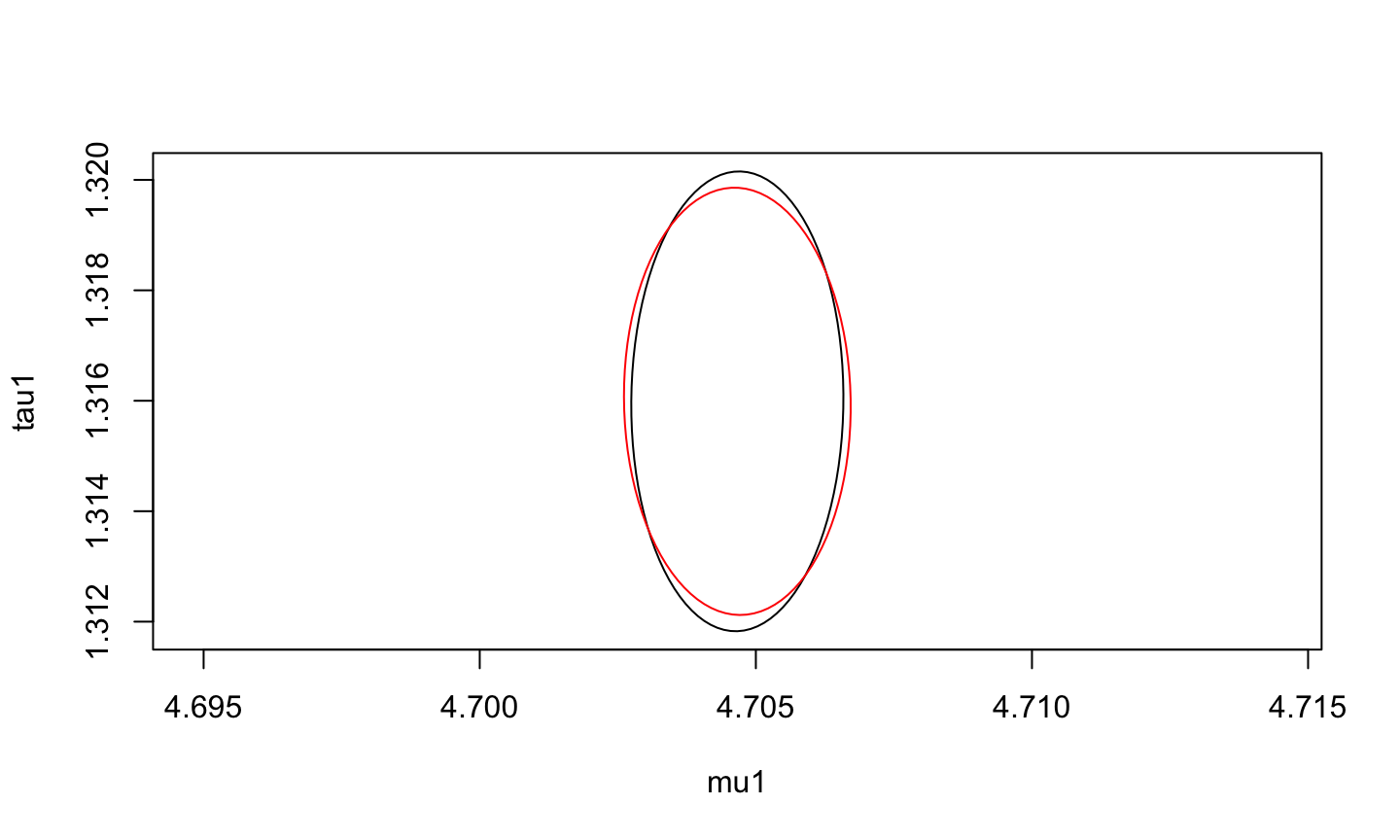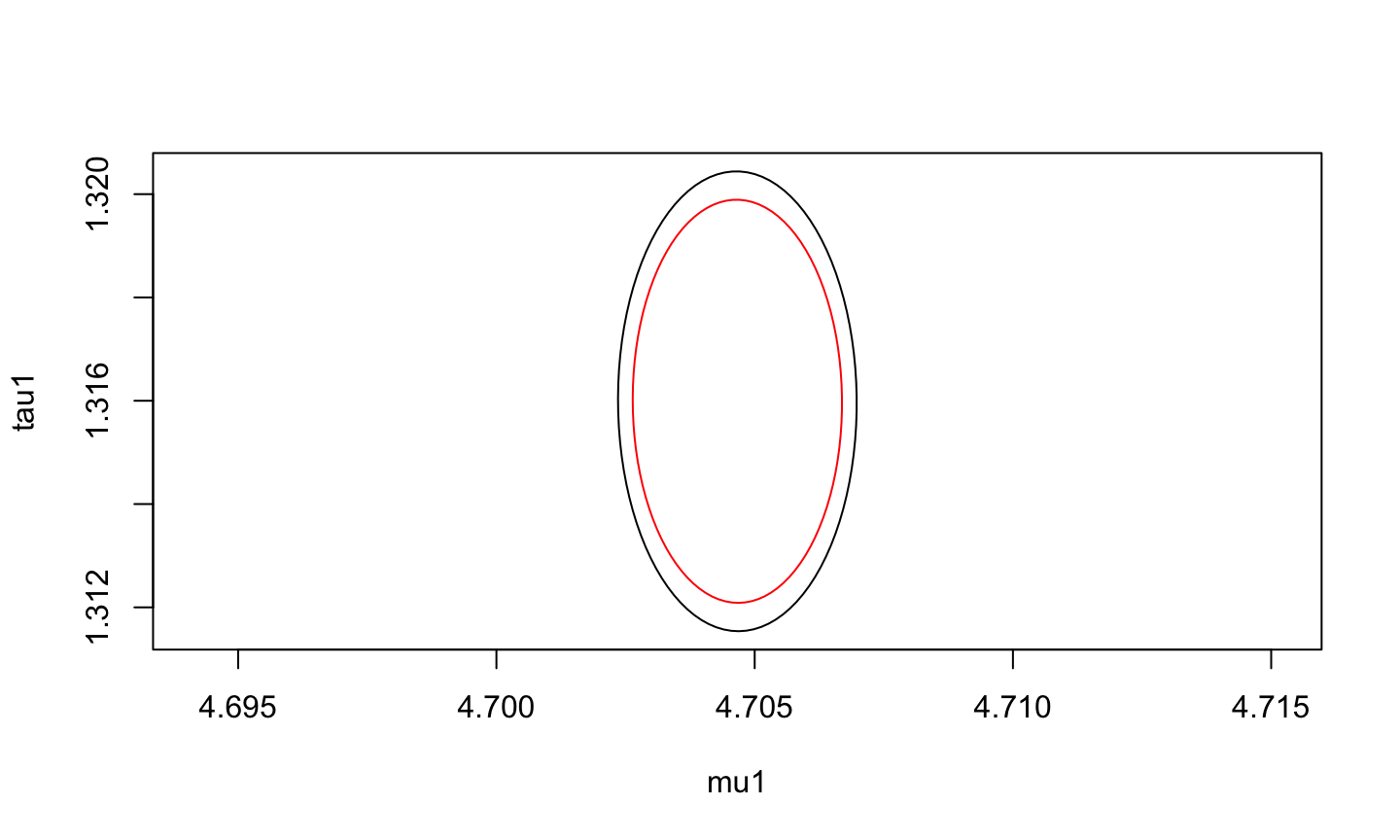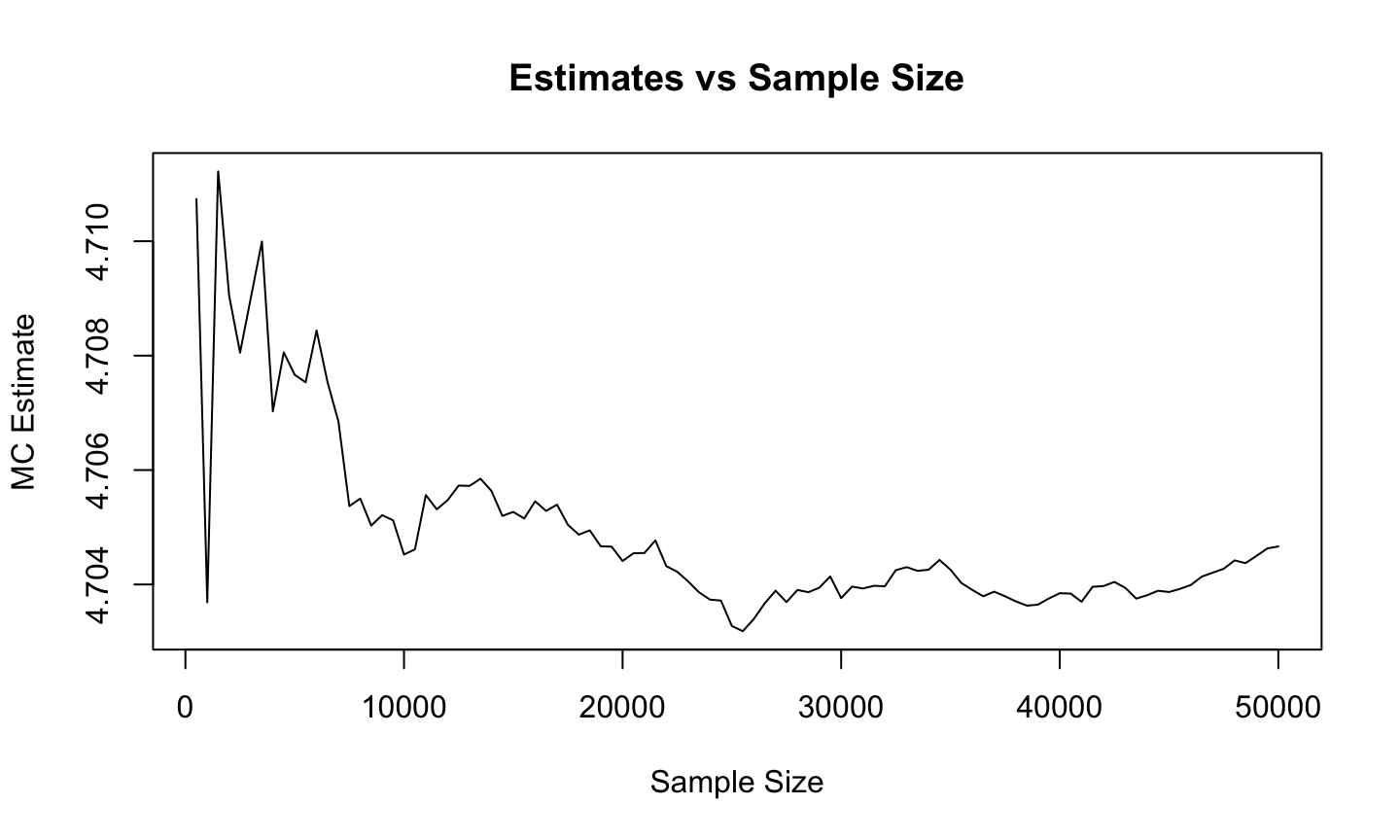## Agenda

• Overview of mcmcse Package
• Diasorin Example
• Confidence Regions
• Effective Sample Size
• Graphical Diagnostics
• Homework

## Overview

• mcmcse provides estimates of Monte Carlo standard errors for MCMC.
• Written by James M. Flegal, John Hughes, Dootika Vats, and Ning Dai.
• Useful when estimating means and quantiles of functions of the MCMC output. In addition to MCMC output, the package can be used for time series and other correlated processes.
• Predominantly useful after MCMC output has been obtained by the user.
• Also provides univariate and multivariate estimates of effective sample size and tools to determine whether enough Monte Carlo samples have been obtained.
• Graphical tools to ascertain the behavior of the Monte Carlo estimates.

## Diasorin Example

• Renal osteodystrophy is a bone disease that occurs when the kidneys fail to maintain proper levels of calcium and phosphorus in the blood. Monitoring patients with loss of kidney function for lower than normal bone turnover aids in managing the disease. A commercially available diagnostic assay, Diasorin, claims to be able to tell patients apart who have low versus normal bone turnover.
• Cross-section of 34 kidney patients from the bone registry at the University of Kentucky were identified as low or normal turnover by other means and then given the commercial assay to determine whether it could correctly identify them. From boxplots a normal sampling model appears untenable due to marked skewness, but boxplots and quantile plots of the log transformed data seem reasonably normal.

## Diasorin Example

set.seed(1)
n1 <- 19
n2 <- 15
low <- c(91, 46,  95,  60, 33, 410, 105, 43, 189,1097, 54,178, 114, 137, 233, 101, 25,70,357)
normal <- c(370, 267, 99,157, 75,1281, 48, 298, 268, 62,804,430,171,694,404)
diasorin <- c(low,normal)
group <- c(rep(1,n1),rep(2,n2))
group1 <- factor(group, 1:2, c('Low','Normal')) 

## Priors

• We elicit independence priors for each population.
• For the low bone turnover group $$\mu_L = \mu_1 \sim N(4.87,0.00288)$$.
• For the normal bone turnover group $$\mu_N = \mu_2 \sim N(5.39,0.00280)$$.
• We consider gamma priors with modes $$\tau_{0,1} = 37.60$$ and $$\tau_{0,2} = 46.53$$.
• Consider $$d = 0.001$$ so $\tau_1 \sim \text{Gamma}(1.0376,0.001) \text{ and } \tau_2 \sim \text{Gamma}(1.04653,0.001).$

## Posterior Sampling

• Use the Gibbs to obtain correlated samples from the posterior.
gibbs <- function(reps, mu, tau, y.bar, s2, n, a, b, c, d){
output <- matrix(0, nrow=reps, ncol=2)
a.gamma <- c+n/2
for(i in 1:reps){
mu.hat <- n * tau / (n * tau + b) * y.bar + b / (n * tau + b) * a
sd.norm <- 1/sqrt(n*tau + b)
mu <- rnorm(1, mu.hat, sd.norm)
b.gamma <- d + ((n-1)*s2 + (y.bar - mu)^2)/2
tau <- rgamma(1, a.gamma, b.gamma)
output[i,] <- c(mu, tau)
}
return(output)
}

## Posterior Sampling

• Simulate for 50,000 iterations.
N <- 50000
y.bar.l <- mean(log(low))
s2.l <- var(log(low))
y.bar.n <- mean(log(normal))
s2.n <- var(log(normal))

sample.l <- gibbs(N, mu=5, tau=1, y.bar = y.bar.l, s2 = s2.l, n = n1,
a=4.87, b=0.00288, c=1.0376, d=0.001)
sample.n <- gibbs(N, mu=5, tau=1, y.bar = y.bar.n, s2 = s2.n, n = n2,
a=5.39, b=0.00280, c=1.04653, d=0.001)

## Posterior Sampling

• For using the mcmcse package the rows of the MCMC output should store each iteration of the algorithm. Thus the output should have $$N$$ rows and $$p$$ columns.
chain <- cbind(sample.l, sample.n)
colnames(chain) <- c("mu1", "tau1", "mu2", "tau2")
head(chain)
##           mu1      tau1      mu2      tau2
## [1,] 4.561362 1.3286643 5.209149 1.1472458
## [2,] 4.538759 1.9654129 5.290550 1.3588665
## [3,] 4.758976 0.9519949 5.562766 1.3602247
## [4,] 4.635779 1.2538313 5.580864 1.6013328
## [5,] 4.642499 1.9271081 5.333285 1.2905995
## [6,] 4.769481 1.0219264 5.534604 0.9064198

## Monte Carlo Estimation

• Discuss estimating two sets of features of interest.
• First, consider $$\nu = \left( E[\mu_1 | y], E[\tau_1 | y], E[\mu_1 | y], E[\tau_1 | y] \right)$$ where the estimator is the Monte Carlo mean $\nu_n = \frac{1}{n} \sum_{i=1}^{n} y_i.$
• In R can can obtain $$\nu_n$$ using the usual colMeans function. If $$p=1$$, then we can use mean instead of colMeans.
colMeans(chain)
##      mu1     tau1      mu2     tau2
## 4.704665 1.315990 5.488848 1.295256

## Monte Carlo Estimation

• Second, consider the ratio $$g(\tau_1, \tau_2) = \tau_2 / \tau_1$$. This is defined in R by creating a function that takes a vector argument.
g <- function(chain){
chain/chain
}
• Then the Monte Carlo estimate for $$g$$ is $\nu_{g,n} = \frac{1}{n} \sum_{i=1}^{n} g(y_i).$
gofy <- apply(chain, 1, g)
mean(gofy)
##  1.088283

## Monte Carlo Estimation

• Thus, to obtain Monte Carlo estimates from MCMC output, the base package is sufficient (generally).
• However, Monte Carlo estimates must be reported with Monte Carlo standard error.
• That is, if the following central limit theorems hold $\sqrt{n} (\nu_n - \nu) \stackrel{d}{\rightarrow} N_p(0, \Sigma)$ and $\sqrt{n} (\nu_{g,n} - g) \stackrel{d}{\rightarrow} N(0, \Sigma_g).$
• Then estimates of $$\Sigma$$ and $$\Sigma_g$$ must be reported.
• Since the samples obtained are correlated, these quantities require more sophisticated tools than usual sample estimators.

## Monte Carlo Standard Error

• In this package, the functions mcse, mcse.mat, mcse.multi, and mcse.initseq estimate the Monte Carlo standard error of $$\nu_{n}$$ or $$\nu_{g,n}$$.
• mcse gives consistent estimates of the square root of $$\Sigma/n$$ (standard error) when $$\Sigma$$ is $$1 \times 1$$.
• mcse.mat gives consistent estimates of the square root of the diagonals of $$\Sigma/n$$.
• mcse.multi gives consistent estimates of $$\Sigma$$.
• mcse.initseq gives asymptotically conservative estimates of $$\Sigma$$ using initial sequence estimators.

## mcmcse Package

library(mcmcse)
## mcmcse: Monte Carlo Standard Errors for MCMC
## Version 1.3-2 created on 2017-07-03.
## copyright (c) 2012, James M. Flegal, University of California, Riverside
##                     John Hughes, University of Colorado, Denver
##                     Dootika Vats, University of Warwick
##                     Ning Dai, University of Minnesota
##  For citation information, type citation("mcmcse").
##  Type help("mcmcse-package") to get started.

## mcmcse Package

mcerror_bm <- mcse.multi(x = chain, method =  "bm",
size = "sqroot", g = NULL, level = .95, large = FALSE)
mcerror_bart <- mcse.multi(x = chain, method =  "bartlett",
size = "cuberoot", g = NULL, level = .95, large = FALSE)
mcerror_tuk <- mcse.multi(x = chain, method =  "tukey",
size = "sqroot", g = NULL, level = .95, large = FALSE)
mcerror_is <- mcse.initseq(x = chain, g = NULL,
level = .95, adjust = FALSE)
mcerror_isadj <- mcse.initseq(x = chain, g = NULL,
level = .95, adjust = TRUE)

## Inputs

• x takes the $$n \times p$$ MCMC data. x can take only numeric entries in the form of a matrix or data frame. The rows of x are the iterations of the MCMC.
• method = ‘‘bm’’, ‘‘bartlett’’, ‘‘tukey’’ calculates the estimate using the batch means method and spectral variance methods with the modified-Bartlett and Tukey-Hanning windows.
• size is the batch size for the bm method and the truncation point for tukey and bartlett methods. size = ‘‘sqroot’’ sets the size as $$\lfloor \sqrt{n} \rfloor$$ and size = ‘‘cuberoot’’ sets it at $$\lfloor n^{1/3} \rfloor$$. An integer value of size less than $$n$$ is also valid.
• g is a function that is applied to each row of x and represents the features of interest of the process. Since here we are interested in only means, g is NULL.

## Inputs

• level is the confidence level of the resulting confidence region. This is required to calculate the volume of the confidence region.
• large is a logical argument. If large is TRUE the volume of the confidence region is the large sample volume obtained using $$\chi^2$$ critical values. By default, volume is calculated using $$F$$ distribution critical values.
• adjust is a logical argument only used for the mcse.initseq function. If adjust is TRUE, the eigenvalues of the initial sequence estimator are increased slightly.

## Outputs

• mcse.multi and mcse.initseq return a list with multiple components.
• cov stores the estimate of $$\Sigma$$ obtained using the method chosen, vol returns the volume to the $$p$$th root of the resulting confidence region, est stores the estimate of g applied on the Markov chain and nsim stores the arguments used to calculate $$\Sigma$$.
• mcse.multi also returns size which indicates the size of batches/truncation, method used, and whether a large sample volume is returned.
• mcse.initseq also returns cov.adj, vol.adj, and whether an adjusted estimator was used (adjust).

## Outputs

mcerror_bm$cov ## [,1] [,2] [,3] [,4] ## [1,] 0.0394643598 0.001375493 0.002379704 -0.0008686777 ## [2,] 0.0013754926 0.185400330 -0.006510334 0.0272105676 ## [3,] 0.0023797040 -0.006510334 0.058530865 -0.0049212774 ## [4,] -0.0008686777 0.027210568 -0.004921277 0.1957994103 mcerror_bart$cov
##               [,1]         [,2]         [,3]          [,4]
## [1,]  0.0457547735 -0.002134084  0.001193809 -0.0003560083
## [2,] -0.0021340836  0.162503174 -0.000597813  0.0028188956
## [3,]  0.0011938092 -0.000597813  0.056796737 -0.0010611261
## [4,] -0.0003560083  0.002818896 -0.001061126  0.1932724203
mcerror_tuk$cov ## [,1] [,2] [,3] [,4] ## [1,] 0.0421549392 0.002086938 0.0005128396 -0.003337617 ## [2,] 0.0020869382 0.176423621 -0.0035763980 0.012953344 ## [3,] 0.0005128396 -0.003576398 0.0532081937 -0.001401419 ## [4,] -0.0033376174 0.012953344 -0.0014014185 0.197610425 mcerror_is$cov
##               [,1]          [,2]          [,3]          [,4]
## [1,]  0.0445562278 -0.0006880102  0.0010955859 -0.0017663968
## [2,] -0.0006880102  0.1653666228 -0.0008183728  0.0025448181
## [3,]  0.0010955859 -0.0008183728  0.0564524841  0.0002125322
## [4,] -0.0017663968  0.0025448181  0.0002125322  0.1947821982
mcerror_isadj$cov.adj ## [,1] [,2] [,3] [,4] ## [1,] 0.0448840031 -0.0009320214 0.0004870070 -0.0011130623 ## [2,] -0.0009320214 0.1656303983 -0.0005832360 0.0019029607 ## [3,] 0.0004870070 -0.0005832360 0.0581606915 -0.0005879199 ## [4,] -0.0011130623 0.0019029607 -0.0005879199 0.1963788341 ## Outputs rbind(mcerror_bm$est, mcerror_bart$est, mcerror_tuk$est,
mcerror_is$est, mcerror_isadj$est)
##           mu1    tau1      mu2     tau2
## [1,] 4.704665 1.31599 5.488848 1.295256
## [2,] 4.704665 1.31599 5.488848 1.295256
## [3,] 4.704665 1.31599 5.488848 1.295256
## [4,] 4.704665 1.31599 5.488848 1.295256
## [5,] 4.704665 1.31599 5.488848 1.295256
##  4.704665
##
## $se ##  0.0008884184 mcse.mat(x = chain, method = "bm", g = NULL) ## est se ## mu1 4.704665 0.0008884184 ## tau1 1.315990 0.0019256185 ## mu2 5.488848 0.0010819507 ## tau2 1.295256 0.0019788856 ## Functionals • In order to estimate $$\nu_{g,n}$$ and $$\Sigma_g$$, we use the R function g we had defined before. • Recall that g should be a function that takes vector inputs. g ## function(chain){ ## chain/chain ## } ## <bytecode: 0x7fbe3ac4e3c8> mcerror_g_bm <- mcse.multi(x = chain, g = g) mcerror_g_is <- mcse.initseq(x = chain, g = g) ## Functionals • Initial Sequence error is larger than batch means, as expected. c(mcerror_g_bm$cov, mcerror_g_is$cov) ##  0.2822692 0.2912683 c(sqrt(mcerror_g_bm$cov/N), sqrt(mcerror_g_is$cov/N)) ##  0.002376002 0.002413580 ## Confidence Regions • Using the function confRegion in the package, the user can create joint confidence regions for two parameters. • Input for this function is the output list from the mcse.multi or mcse.initseq function. • Function uses the attributes cov, est, and nsim from the output list. • If the mcse.initseq is input and adjust = TRUE had been used, then cov.adj is used instead of cov. • mcse.multi also uses the attribute size. ## Confidence Regions plot(confRegion(mcerror_bm, which = c(1,2), level = .90), type = 'l', asp = 1, xlab="mu1", ylab="tau1") lines(confRegion(mcerror_bart, which = c(1,2), level = .90), col = "red")## Confidence Regions • To determine the effect of the confidence level, we draw two regions with difference confidence levels. We use mcse.initseq this time. plot(confRegion(mcerror_is, which = c(1,2), level = .95), type = 'l', asp = 1, xlab="mu1", ylab="tau1") lines(confRegion(mcerror_is, which = c(1,2), level = .90), col = "red")## Effective Sample Size • Reporting $$p \times p$$ covariance matrix estimates is impractical and uninterpretable. • The motivation of estimating Monte Carlo standard error is to ensure that said error is small. • Essentially the idea behind estimating effective sample size and ensuring that the estimated effective sample size is larger than a prespecified lower bound. ## Effective Sample Size • Before sampling the Markov chain, the user is advised to used the function minESS to ascertain what is the minimum effective sample size needed for stable analysis. • p is the dimension of the estimation problem. • alpha is the confidence level. • eps is the tolerance level. Default is .05. Reasonable levels are anywhere from .01 to .05. The smaller the tolerance, the larger the minimum effective samples. eps represents a tolerance level relative to the variability in the target distribution. It is akin to the idea of margin-of-error. minESS(p = 4, alpha = .05, eps = .05) ## minESS ## 8431 minESS(p = 1, alpha = .05, eps = .05) ## minESS ## 6146 ## Effective Sample Size • minESS is independent of the Markov chain or process, and is only a function of the p, alpha, and eps. • User should find minESS and then sample their process until the required minimum samples are achieved. • Alternatively, we often don’t have to luxury of obtaining a lot of samples, and reaching a minimum effective sample size os not possible. In such a scneario, it is useful to know the eps tolerance level the number of estimated effective samples correspond to. ## Effective Sample Size • If we can only obtain 1000 effective samples, minESS(p = 4, alpha = .05, ess = 1000) ## Epsilon ## 0.1451773 minESS(p = 1, alpha = .05, ess = 1000) ## Epsilon ## 0.123959 ## Effective Sample Size • multiESS and ess are two functions that calculate the effective sample size of a correlated sample. • ess calculations are component-wise while multiESS utilizes the multivariate nature of the problem. ess(chain) ## mu1 tau1 mu2 tau2 ## 55801.49 44545.19 49721.07 50076.02 multiESS(chain) ##  50237.53 multiESS(chain, covmat = mcerror_bart$cov)
##  50233.54

## Effective Sample Size

• Since ess produces a different estimate for each component, conservative practice dictates choosing the smallest of the values.
• multiESS returns one estimate of the effective sample size based on the whole sample.
• Function calls mcse.multi function to obtain a batch means estimate of $$\Sigma$$. User can provide another estimate of $$\Sigma$$ using the covmat argument.
• Can compare effective sample size to minimum effective samples, and adjust our simulation length if necessary. For example, by looking at the ratio of the Monte Carlo samples size and multiESS.

## Graphical Diagnostics

• The function estvssamp plots the Monte Carlo estimates versus the sample size for a component of the MCMC output. This plot indicates whether the Monte Carlo estimate has stabilized.
estvssamp(chain[,1])## Graphical Diagnostics

• Additionally, if $$p$$ is not too small, due to the CLT and an estimate of $$\Sigma$$ using the mcse.multi function, a QQ plot of the standardized estimates gives an idea of whether asymptopia has been achieved.
• However, $$p=4$$ in our example so we need another …

## Diasorin Summary

mu1 <- sample.l[,1]
tau1 <- sample.l[,2]
mu2 <- sample.n[,1]
tau2 <- sample.n[,2]
mu.diff <- mu2-mu1
tau.ratio <- tau2/tau1
post.sum <- function(vec){
return(c(mean(vec), sd(vec), quantile(vec, probs=c(0.25, .5, .975))))
}
sum.table <- rbind(post.sum(mu1), post.sum(mu2), post.sum(mu.diff), post.sum(tau1),
post.sum(tau2), post.sum(tau.ratio))
rownames(sum.table) <- c("mu1", "mu2", "mu2-mu1", "tau1", "tau2", "tau2/tau1")
colnames(sum.table) <- c("mean", "sd", "2.5%", "50%", "97.5%")
round(sum.table, 3)
##            mean    sd  2.5%   50% 97.5%
## mu1       4.705 0.210 4.566 4.705 5.117
## mu2       5.489 0.241 5.332 5.489 5.968
## mu2-mu1   0.784 0.319 0.572 0.784 1.415
## tau1      1.316 0.406 1.024 1.274 2.227
## tau2      1.295 0.443 0.976 1.246 2.299
## tau2/tau1 1.088 0.543 0.711 0.975 2.439

## Homework

• Use the mcmcse package to add MCSEs to the summary statistics in sum.table.
• Need to use the mcse.q function for quantiles.
• What method and size did you use? Why?
• Create a plot using the mcmcse package of your choosing. Explain carefully what the plot is illustrating.